# The pH ScalePage 1

#### WATCH ALL SLIDES

Slide 1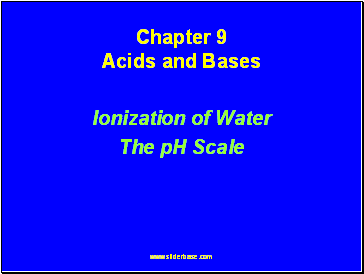LecturePLUS Timberlake

1

Chapter 9 Acids and Bases

Ionization of Water

The pH Scale

Slide 2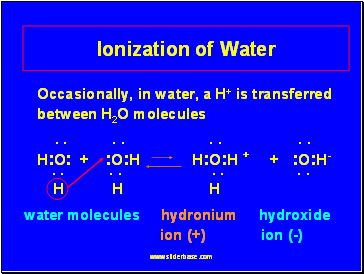## Ionization of Water

LecturePLUS Timberlake

2

Occasionally, in water, a H+ is transferred between H2O molecules

. . . . . . . .

H:O: + :O:H H:O:H + + :O:H-

. . . . . . . .

H H H

water molecules hydronium hydroxide ion (+) ion (-)

Slide 3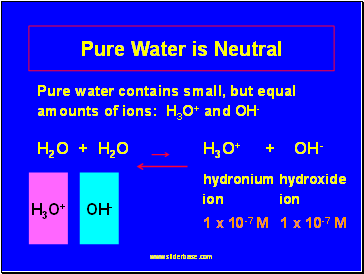## Pure Water is Neutral

LecturePLUS Timberlake

3

Pure water contains small, but equal amounts of ions: H3O+ and OH-

H2O + H2O H3O+ + OH-

hydronium hydroxide ion ion

1 x 10-7 M 1 x 10-7 M

H3O+

OH-

Slide 4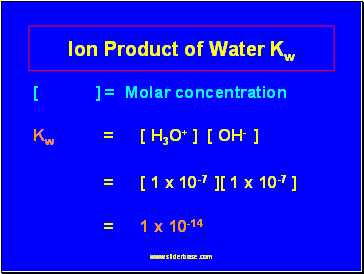## Ion Product of Water Kw

LecturePLUS Timberlake

4

[ ] = Molar concentration

Kw = [ H3O+ ] [ OH- ]

= [ 1 x 10-7 ][ 1 x 10-7 ]

= 1 x 10-14

Slide 5## Acids

LecturePLUS Timberlake

5

Increase H+

HCl (g) + H2O (l) H3O+ (aq) + Cl- (aq)

More [H3O+] than water > 1 x 10-7M

As H3O+ increases, OH- decreases

[H3O+] > [OH-]

H3O+

OH-

Slide 6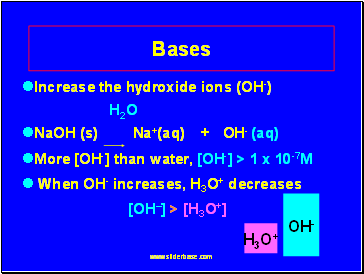## Bases

LecturePLUS Timberlake

6

Increase the hydroxide ions (OH-)

H2O

NaOH (s) Na+(aq) + OH- (aq)

More [OH-] than water, [OH-] > 1 x 10-7M

When OH- increases, H3O+ decreases

[OH] > [H3O+]

H3O+

OH-

Slide 7LecturePLUS Timberlake

7

Using Kw

The [OH- ] of a solution is 1.0 x 10- 3 M. What is the [H3O+]?

Kw = [H3O+ ] [OH- ] = 1.0 x 10-14

[H3O+] = 1.0 x 10-14

[OH-]

[H3O+] = 1.0 x 10-14 = 1.0 x 10-11 M

1.0 x 10- 3

Slide 8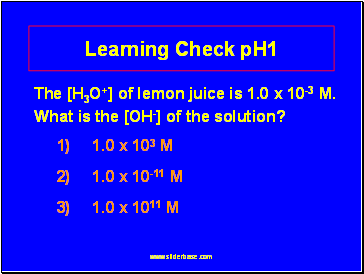LecturePLUS Timberlake

8

Learning Check pH1

The [H3O+] of lemon juice is 1.0 x 10-3 M. What is the [OH-] of the solution?

1) 1.0 x 103 M

2) 1.0 x 10-11 M

3) 1.0 x 1011 M

Slide 9LecturePLUS Timberlake

9

Solution pH1

The [H3O+] of lemon juice is 1.0 x 10- 3 M. What is the [OH-]?

[OH- ] = 1.0 x 10 -14 = 1.0 x 10-11 M

1.0 x 10 - 3

Slide 10## Using the Calculator

LecturePLUS Timberlake

10

1.0 x 10 -14

Go to page:
1  2  3  4